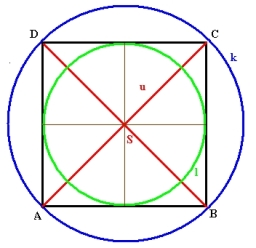# Circumference 56291

Calculate the circumference of a circle circumscribed by a right triangle with squares 10 cm and 15 cm long.

x =  56.64 cm

### Step-by-step explanation:Did you find an error or inaccuracy? Feel free to write us. Thank you!

Tips to related online calculators
Pythagorean theorem is the base for the right triangle calculator.

#### Level of the problem:

We encourage you to watch this tutorial video on this math problem: